Effective ICSE Class 9 Maths Solutions S Chand Chapter 11 Rectilinear Figures Ex 11(B) can help bridge the gap between theory and application.

## S Chand Class 9 ICSE Maths Solutions Chapter 11 Rectilinear Figures Ex 11(B)

Question 1.
(i) If in a parallelogram, the diagonals are equal in length and intersect at right angles, prove that the parallelogram will be a square.
(ii) Prove that in a rectangle, the diagonals are of equal lengths.
Solution:
(i) Given : ABCD is a parallelogram in which diagonal AC = diagonal BD and AC and BD intersect each other at O at right anglesTo prove : ABCD is a square
AB = AB (common)
AC = BD (given)
BC = AD (opposite sides of || gm)
∴ ∆ABC ≅ ∆ADC (SSS axiom)
But ∠ABC + ∠B AD = 180° (co-interior angles)
∴ ∠ABC = ∠BAD = 90°
Again in ∆AOB and ∆BOC
∠AOB = ∠BOC (each 90°)
BO = BO (common)
AO = OC (∵ diagonals bisect each other)
∴ ∆AOB ≅ ∆BOC (SAS axiom)
∴ AB = BC
But AB = CD and BC = AD (opposite sides of ||gm)
∴ AB = BC = CD = DA
and each angle is 90°
Hence ABCD is a square
Hence proved.

(ii) Given : ABCD is a rectangle and AC and BD are its diagonals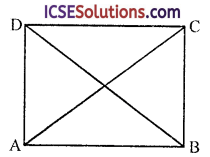To Prove: AC = BD
AB = AB (common)
BC = AD (opposite sides of rectangle)
∴ ∆ABC ≅ ∆ADB (SAS axiom)
AC = BD (c.p.c.t.)
Hence proved.

Question 2.
In the figure, ABCD is a parallelogram. M is the mid-point of AC; X, Y are points on AB and DC respectively such that AX = CY.
Prove that:
(i) ∆AXM ≅ ∆CYM
(ii) XMY is a straight line.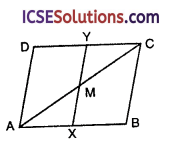Solution:
Given : In parallelogram ABCD, AC is its diagonal X and Y are the mid-points of AB and CD respectively
M is mid-point of AC and AX = CY
To prove :
(i) ∆AXM ≅ ∆CYM
(ii) XMY is a straight line
Construction : Join XM and YM
Proof: In ∆AMX and ∆CMY
AX = CY (given)
AM = CM (M is mid-point of AC)
and ∠YCM = ∠MAX (alternate angles)
∴ ∆AMX = ∆CMY (SSA axiom)
∴ ∠AMX = ∠CMY
But these are vertically opposite angles
∴ XMY is a straight line
Hence proved.Question 3.
Prove that :
(i) A diagonal of a square makes an angle of 45° with the side of the square.
(ii) The diagonals of a rhombus are at right’ angles.
(iii) A diagonal of a rhombus bisects the angles at vertices.
Solution:
Given :
(i) In a square ABCD, BD is its diagonalTo prove : ∠ABD = 45°
Proof: In ∆ABD and ABCD
AB = DC (sides of square)
AD = BC (sides of square)
BD = BD (common)
∴ ∆ABD ≅ ∆BCD (SSS axiom)
∴ ∠ABD =∠CBD (c.p.c.t.)
But ∠ABD + ∠CBD = 90°
∴ ∠ABD = $$\frac { 90° }{ 2 }$$ = 45°
Hence diagonal BD makes an angle of 45° with the side of the square

(ii) Given : In rhombus ABCD its diagonals AC and BD bisect each other at O
To prove : ∠AOB = ∠BOC = ∠COD = ∠DOA – 90°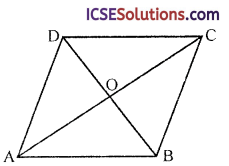Proof: In ∆AOB and ∆COB
AQ = OC (diagonals bisect each other)
BO = BO (common)
AB = BC (sides of rhombus)
∴ ∆AOB = ∆COB (SSS axiom)
∴ ∠AOB ≅ ∠COB (c.p.c.t.)
But ∠AOB + ∠COB = 180° (Linear pair)
∴ ∠AOB = ∠COB = 90°
Similarly we can prove that
∠COD = ∠DOA = 90°
Hence diagonals of a rhombus bisect each other at right angles

(iii) Given : In rhombus ABCD, AC is its diagonal
To prove : AC, bisect ∠A and ∠CAB = CD (opposite sides of rhombus)
AC = AC
(common)
∴ ∆ABC ≅ ∆ADC (SSS axiom)
and ∠ACB = ∠ACD (c.p.c.t.)
Hence diagonal of a rhombus bisects the angles at the vertices.Question 4.
In the figure, PQRS is a parallelogram. QU and ST are perpendicular on the diagonal PR. Prove that
(i) ∆STR ≅ ∆QUP
(ii) ST = QUSolution:
Given : PQRS is a parallelogram in which PR is its diagonal ST ⊥ PR and QU ⊥ PR
To prove :
(i) ∆STR ≅ ∆QUP
(ii) ST = QU
Proof: In ∆STR and ∆QUP
PS = SR (Opposite sides of parallelogram)
∠T = ∠U (each 90°)
∠SPT = ∠URQ (alternate angles)
(i) ∴ ∆STR ≅ ∆QUP (AAS axiom)
(ii) ∴ ST = QU (c.p.c.t.)

Question 5.
In the figure, PQRS is a parallelogram PO and QO are respectively the angle bisectors of ∠P and ∠Q. Line LOM is drawn parallel to PQ. Prove that
(i) PL = QM
(ii) LO = OM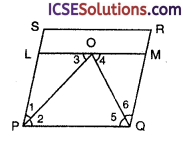Solution:
Given : In ||gm ABCD, PO and QO are the angle bisectors of ∠P and ∠Q respectively meeting each other at O. LOM is drawn parallel to PQ through O
To prove :
(i) PL = QM
(ii) LO = OM
Proof: ∵ PO is the bisector of ∠P
∴ ∠1 = ∠2
Similarly QO is the bisector of ∠Q
∴ ∠5 = ∠6
∵ LM || PQ
LPQM is a parallelogram
and ∠3 = ∠2 = ∠1 (alternate angles)
and ∠4 = ∠5 = ∠6
(i) LP = QM (∵ LPQM is a parallelogram)
(ii) PL = LO (∵ ∠1 = ∠3)
and OM = OM (∵ ∠4 = ∠6)
But PL = OM (proved)
∴ LO = OM
Hence proved.

Question 6.
(i) ABCD is a parallelogram. L and M are points on AB and DC respectively and AL = CM. Prove that LM and BD bisect each other.(ii) ABCD is a parallelogram. AB is produced to E such that BE = AB. Prove that ED bisects BC.Solution:
(i) Given : In ||gm ABCD, BD is its diagonal L and M are points on AB and CD respectively such that AL = CM
To prove : LM and BD bisect each other i.e. BO = OD and LO = OM
Proof : In ||gm ABCD,
AB = CD
But AL = CM
∴ AB – AL = CD – CM
⇒ LB = MD
Now in ∆LOB and ∆DOM
LB = MD (proved)
∠LBO = ∠MDO (alternate angles)
∠LOB = ∠DOM
(vertically opposite angles)
∴ ∆LOB ≅ ∆DOM (AAS axiom)
∴ OB = OD and OL = OM(c.p.c.t.)
Hence BD and LM bisect each other

(ii) Given : In a parallelogram ABCD, AB is produced to E such that BE = AB
To prove : ED bisects BC
Proof:
∵ AB = DC (opposite sides of ||gm)
and AB = BE (given)
∴ DC = BE
Now in ∆BOE and ∆COD
BE = DC (Proved)
∠BOE = ∠COD (vertically opposite angles)
∠BEO = ∠CDO (alternate angles)
∴ ∆BOE ≅ ∆COD (AAS axiom)
∴ BO = OC (c.p.c.t.)
Hence DE bisects BC

Question 7.
(i) The diagonals of a parallelogram intersect at O. A line through O intersects AB at X and DC at Y. Prove that OX = OY.
(ii) In atriangle ABC, median AD is produced to X, such that AD = DX. Prove that ABXC is a parallelogram.
Solution:
(i) Given : In parallelogram ABCD diagonals AC and BD intersect each other at O
A line XOY is drawn which meets AB in X and CD in Y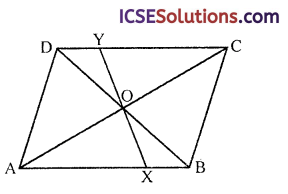To prove : OX = OY
Proof: In ∆XOB and ∆YOD
OB = OD (diagonals bisect each other)
∠BOX = ∠DOY
(vertically opposite angles)
∠OBX = ∠ODY (alternate angles)
∴ ∆XOB ≅ ∆YOD (AAS axiom)
∴ OX = OY (c.p.c.t.)
Hence proved.

(ii) Given : In AABC, AD is the median which is produced to X such that DX = AD BX and XC are joined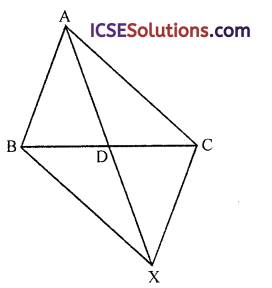To prove : ABXC is a parallelogram
BD = DC (D is mid-point)
(vertically opposite angles)
∴ ∆ADB ≅ ∆XDC (SAS axiom)
∴ AB = CX (c.p.c.t.)
But these are alternate angles
∴ AB || XC
∵ AB = XC and AB || XC
∴ ABXC is a parallelogram
Hence proved.

Question 8.
In a parallelogram ABCD, the bisector of ∠A meets DC in E and AB = 2AD. Prove that (i) BE bisects ∠B, (ii) ∠AEB is a right angle.
Solution:
Given : (i) In parallelogram ABCD, AE is the bisector of ∠A which meets CD in E, AB = 2AD
EB is joinedTo prove :
(i) BE bisects ∠E
(ii) ∠AEB is a right angle
Construction : Take F, a mid-point of AB. Join EF
Proof :
∵ AB = 2AD and F is mid-point of AB
∵ ∠1 = ∠2 (AE is the bisector of ∠A)
∴ AFED is a rhombus
AD || EF || CD and E is mid-point of CD
∴ FBCE is also a rhombus
whose BE is diagonal
But diagonal of a rhombus bisects the angles at the vertices
∴ BE bisects ∠E(ii) ∵ ∠1 = ∠2 and ∠3 = ∠4
But ∠A + ∠B = 180° (co-interior angles)
2∠2 + 2∠3 = 180°
⇒ ∠2 + ∠3 = 90° (dividing by 2)
In ∆AEB,
∵ ∠2 + ∠3 + ∠AEB = 180° (angles of a triangle)
⇒ 90° + ∠AEB = 180°
⇒ ∠AEB = 180° – 90°
⇒ ∠AEB = 90°
Hence ∠AEB is a right angle

Question 9.
(i) In the figure, ABCD is a ||gm. X is the mid-point of AD and Y is the mid-point of BC. Prove that AYCX is a ||gm and that XY and BD bisect each other.(ii) In the figure, ABCD is a ||gm. BM bisects ∠ABC, and DN bisects ∠ADC. Prove that:
(i) BNDM is a ||gm,
(ii) BM = DN.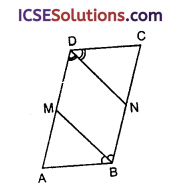(iii) In the figure, BM bisects ∠B and AN bisects ∠A of ||gm ABCD. Prove that :
(i) MN = CD
(ii) ABNM is a rhombusSolution:
(i) Given : In ||gm ABCD,
X and Y are mid-points of the side AD and BC respectively. BD is its diagonal. AY and CX are joined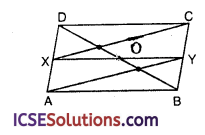To prove :
(i) ATCX is a ||gm
(ii) XY and BD bisect each other
Proof:
∵ AD || BC (opposite sides of ||gm)
⇒ AX || CY
But X and Y are mid-points of AD and BC
AX = CY (half of equal sides)
AXCY is a ||gm

(ii) In ∆XOD and ∆BOY
XD = BY (half of equal sides)
∠XOD = ∠BOY
(vertically opposite angles) ∠ADO = OBY (alternate angles)
∴ ∆XOD ≅ ∆BOY (AAS axiom)
∴ XO = OY (c.p.c.t.)
and DO = OB (c.p.c.t.)
Hence XY and BD bisect each other
Hence proved.

(ii) Given : In ||gm ABCD,
BM is the bisector of ∠B and DN is the bisector of ∠D
To prove :
(i) BNDM is a ||gm
(ii) BM = DN
Proof: ∵ Bisector of ∠ABC meets AD at M
∴ M is mid-point of AD
Similarly DN is the bisector of ∠ADC
∴ N is mid-point of BC
∴ MD || BN (∵ AD || BC)
and DM = BN (half of equal sides)
∴ BNDM is a ||gm
∴ BM = DM (opposite sides of || gm BNDM)
Hence proved.

(iii) Given : In || gm ABCD, BM is the bisector of ∠B and AN is the bisector of ∠ATo prove :
(i) MN = CD
(ii) ABNM is a rhombus
Proof :∠A + ∠B = 180° (co-interior angles)
∵ AN and BM are the bisectors of ∠A and ∠B respectively
∴ $$\frac { 1 }{ 2 }$$ ∠A + $$\frac { 1 }{ 2 }$$ ∠B = 90°
⇒ ∠OAB + ∠OBA = 90°
∴ In ∆AOB,
∠AOB = 180° – 90° = 90°
∴ AN and BM are perpendicular to each other
Now in ∆AOB and ∆MON
AB = MN (opposite sides of ||gm)
∠AOB = ∠MON
(vertically opposite angles)
∠BAN = ∠ANM (alternate angle)
∴ ∆AOB ≅ ∆MON (AAS axiom)
∴ AO = ON and BO = OM
∵ Diagonals BM and AN bisect each other at right angles
∴ ABNM is a rhombus

Question 10.
A transversal cuts two parallel lines at A and B. The two interior angles at A are bisected and so are the two interior angles at B; the four bisectors form a quadrilateral ACBD. Prove that:
(i) ACBD is a rectangle.
(ii) CD is parallel to the original parallel lines.
Solution:
Given : Two parallel line PQ and RS and a transversal LM intersects them at A and B respectively
The bisectors of interipr angles at A and B, meet each other at C and D as shown in the figure fonning a quadrilateral ABCD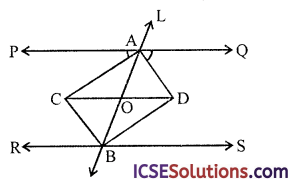To prove :
(i) ACBD is a rectangle
(ii) CD is parallel to the original parallel lines PQ and RS
Construction : Join CD
Proof:
∵ (i) AC and BD are the bisectors of ∠PAB and ∠ABS and AC || BD
ACBD is a parallelogram
Now ∠PAB + ∠ABR = 180°
∴ $$\frac { 1 }{ 2 }$$ ∠PAB +$$\frac { 1 }{ 2 }$$ ∠ABR = 90°
⇒ ∠CAB + ∠CBA = 90°
In ∆ABC,
∠ACB = 90°
∴ A parallelogram with each angle a right angle is a rectangle
Hence ACBD is a rectangle

(ii) ∵ Diagonals of a rectangle are equal and bisect each other
Diagonal AB and CD of rectangle ACBD bisect each other at O
∴ OA = OC
∴ ∠OAC = ∠ACO
⇒ ∠CAP = ∠ACO
But these are alternate angles
∴ CD || PQ or RS

Question 11.
In the figures,(i) BY bisects ∠B of AABC. Prove that B∠YX is a rhombus.
(ii) HJKL is a square, HO = HX. Prove that ∠HOX = 3∠XOJ.
(iii) ABCD is a || gm. ABML and ADXY are squares. Prove that ACXM is isosceles.
(iv) ABCD and ALMN are squares. Prove that BL = DN.
Solution:
(i) Given : In ∆ABC, BY is the bisector of ∠B meeting AC at Y
Through Y. YX || AB and YZ || BC are drawn
To prove : B∠YX is a rhombus
Proof: ∵ XY || BA or BZ
and YZ || BC or BX∴ B∠YX is a parallelogram
But BY is the diagonal which bisects ∠B (given)
∴ B∠YX is a rhombus

(ii) Given : HJKL is a square in which diagonals
HK and JL intersect each other at O
X is a point on HJ such that HX = HO
XO is joined
To prove : ∠HOX = 3∠XOJ
Proof: ∵ Diagonals of square HJKL bisect each other at right angle at O
∴ ∠HOJ = 90° and ZKHJ or ∠OLIX = 45°
∵ In AOHX
OH = HX (given)
∴ ∠HOX = ∠HXO
(angles opposite to equal side)
But ∠OHX + ∠HOX + ∠HXO = 180°
(angles of a triangle)
⇒ 45° + ∠HOX + ∠HOX = 180°
⇒ 2∠HOX = 180° – 45°= 135°
⇒∠HOX= $$\frac { 135 }{ 2 }$$ = 67$$\frac { 1° }{ 2 }$$ … (i)
But ∠XOT = ∠HOJ = ∠HOX
90° – 67$$\frac { 1° }{ 2 }$$ = 22$$\frac { 1° }{ 2 }$$ … (ii)
From (i) and (ii)
∠HOX = 67 $$\frac { 1° }{ 2 }$$ = 3 x 22$$\frac { 1° }{ 2 }$$
= 3∠XOJ
Hence proved.(iii) Given : In the figure,
ABCD is a ||gm
ABL and ∆DXY are squares
To prove : ∆CXM is an isosceles triangle
Construction : Join MC, MX and CX
Proof: In parallelogram ABCD
AB = CD and BC = AD (opposite sides)
and ∠ABC = ∠CDA and ∠BCD = ∠BAD (opposite angles)
∠MBC = ∠MBA + ∠ABC = 90° + ∠ABC … (i)
∠CDX = ∠CDA + ∠ADX = ∠ABC+ 90° … (ii)
(∵ ∠CDA = ∠ABC)
∴ From (i) and (ii),
∠MBC = ∠CDX
Now in ∆BMC and ∆DCX
BM = BA = CD (given)
BC = AD = DX (given)
and ∠MBC = ∠CDX (proved)
∆BAC ≅ ∆DCX (SAS axiom)
∴ CM = CX
Hence ACMX is an isosceles triangle

(iv) Given : ABCD and ALMN are two squares as shown in the figure
To prove : BL = DN
Construction : Join DM and BL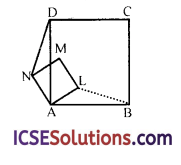AD = AB (sides of square ABCD)
AN = AL (sides of square ALMN)
∠DAN = ∠LAB (each = 90° – ∠LAD)
∴ ∆ADN ≅ ∆ALB (SAS axiom)
∴ DN = BL (c.p.c.t.)
Hence proved.

Question 12.
(i) Prove that the bisectors of any two adjacent angles of a parallelogram are at right angles.
(ii) In the figure, PQRS is a parallelogram with bisectors PA, QD, RC and SB respectively of angles P, Q, R and S. Show that ABCD is an rectangle.Solution:
(i) Given : In ||gm ABCD, AE and BE are the bisectors of adjacent ∠A and ∠B which meet at ETo prove : ∠E is a right angle
Proof: ∵ AE is the bisector of ∠A
∴ ∠EAB = $$\frac { 1 }{ 2 }$$ ∠A
Similarly BE is the bisector of ∠B
∴ ∠EBA = $$\frac { 1 }{ 2 }$$ ∠B
But ∠A + ∠B = 180° (co-interior angles)
∴ $$\frac { 1 }{ 2 }$$ ∠A + $$\frac { 1 }{ 2 }$$ ∠B = 90°
⇒ ∠EAB + ∠EBA = 90°
But in ∆AEB
∠EAB + ∠EBA + ∠AEB = 180°
(sum of angles of a triangle)
⇒ 90° + ∠AEB = 180°
⇒ ∠AEB = 180° -90° = 90°
∴ ∠E is a right angle

(ii) Given : In ||gm ABCD, PA, QD, RC and SB are the bisectors of ∠P, ∠Q, ∠R and ∠S respectively which meet each other at A, C, D and B respectively forming a quad. ABCD
To prove : ABCD is a rectanlge
Proof:
∵ PD and QD are the bisectors of two adjacent angles ∠P and ∠Q respectively
∴ ∠D = 90°
Similarly ∠B = 90°
and PA and SA are the bisectors of ∠P and ∠S respectively
∴ ∠A = 90°
Similarly ∠C = 90°
∴ The quad. ABCD has its each angle equal to 90°
∴ ABCD is a rectangle
Hence proved.

Question 13.
In the figure, ABCD is a parallelogram and X is the midpoint of BC. The line AX produced meets DC produced at Q. The parallelogram ABPQ is completed. Prove that:
(i) ∆ABX ≅ ∆QCX
(ii) DC = CQ = QPSolution:
Given : ABCD is a ||gm and X is mid-point of BC
AX is joined and produced to meet BC produced at Q. The ||gm ABPQ is completed as shown in the figure
To prove :
(i) ∆ABX ≅ ∆QCX
(ii) DC = CQ = QP
Proof: In ∆ABX and ∆QCX
BX = XC (∵ Q is mid-point of BC)
∠AXB = ∠CXQ
(vertically opposite angles)
∠ABX = ∠XCQ (alternate angles)
∴ ∆ABX ≅ ∆QCX (AA side axiom),
∴ AB = CQ (c.p.c.t.)
But AB = DC and AB = QP
(opposite sides of ||gms)
∴ DC = CQ = QP
Hence proved.

Question 14.
ABCD is a rhombus with P, Q, R as mid-points of AB, BC and CD. Prove that PQ ⊥ QR.
Solution:
Given : P, Q and R are the mid points of the sides AB, BC and CD of rhombus ABCD PQ and QR are joinedTo prove : PQ ⊥ QR
Construction : Join diagonals AC and BD of the rhombus ABCD
Proof: In ∆ABC,
P and Q are mid-points of AB and BC respectively
∴ PQ || AC … (i)
Similarly in ∆BCD,
Q and R the mid-points of BC and CD respectively
∴ QR || BD … (ii)
But AC and BD bisect each other at right angles
∴ PQ ⊥ QR

Question 15.
ABCD is a rhombus. RABS is a straight line such that RA = AB = BS. Prove that RD and SC when produced meet at right angles.
Solution:
Given : In a rhombus ABCD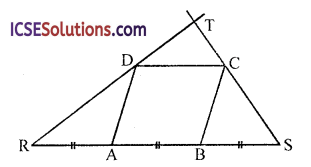RABS is a straight line such that RA = AB = RS
RD and SC are joined and produced to meet atT
To prove : ∠T = 90°
∴ ∠DRA = ∠RDA
and Ex. ∠DAB = ∠DRA + ∠RDA = ∠DRA + ∠DRA = 2∠DRA … (i)
Similarly in ∆BSC,
BS = AB = BC
∴ ∠BCS = ∠BSC
and Ext. ∠ABC = ∠BCS + ∠BSC
= ∠BSC + ∠BSC = 2∠BSC … (ii)
But in rhombus ABCD,
∠DOB + ∠ABC = 180° (co-interior angles)
⇒ 2∠DRA + 2∠BSC = 180°
∠DRA + ∠BSC = 90° (dividing by 2)
Now in ∆RTS,
∠DRA + ∠BSC = 90°
or ∠TRS + ∠RST = 90°
∴ ∠RTS = 180°- 90° = 90°
Hence ∠T = 90°